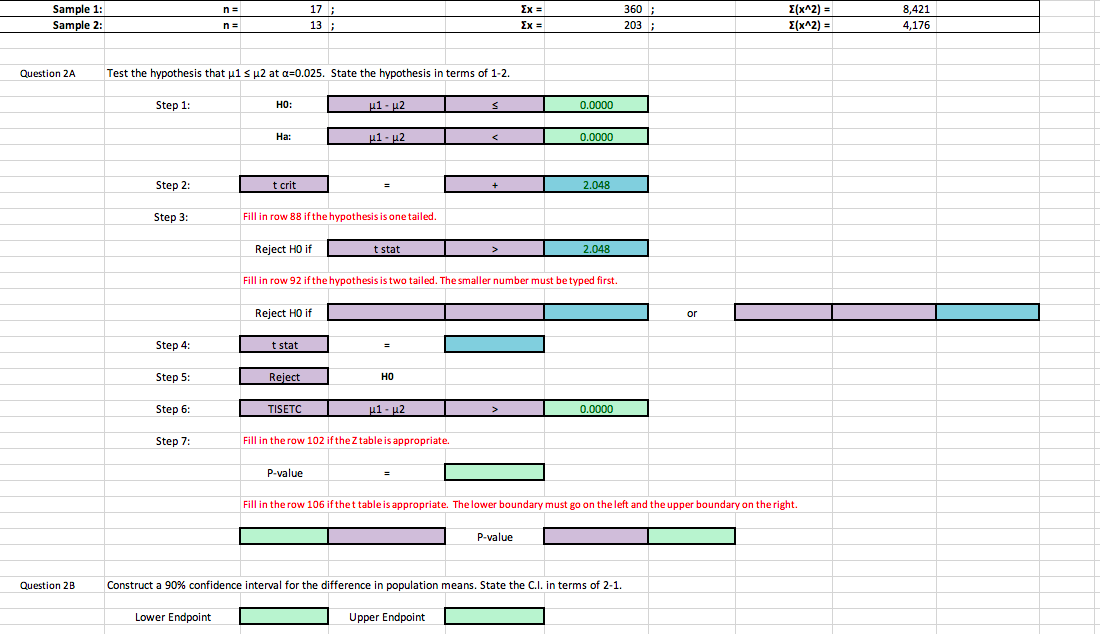# Sample 1:Sample 2:1713360038,4214,176E(x^2)Question 2ATest the hypothesis that μ1μ2 at α-0.025. State the hypothesis in terms of 1-2.Step 1:HO:0.0000Ha:0.0000Step 2:t crit2.048Step 3:Fill in row 88 ifthe hypothesis is one tailedReject HO ift stat2.048Fill in row 92 if the hypothesis is two tailed. The smaller number must be typed first.Reject HO iforStep 4:Step 5:Step 6:Step 7:t statReiectHO0.0000Fill in the row 102 ifthe Z table is appropriate.P-valueFill in the row 106 ifthet table is appropriate. The lower boundary must go on the left and the upper boundary on the right.P-valueQuestion 2BConstruct a 90% confidence interval for the difference in population means. State the C.1, in terms of 2-1.Lower EndpointUpper Endpoint

Question
5 viewshelp_outlineImage TranscriptioncloseSample 1: Sample 2: 17 13 360 03 8,421 4,176 E(x^2) Question 2A Test the hypothesis that μ1 μ2 at α-0.025. State the hypothesis in terms of 1-2. Step 1: HO: 0.0000 Ha: 0.0000 Step 2: t crit 2.048 Step 3: Fill in row 88 ifthe hypothesis is one tailed Reject HO if t stat 2.048 Fill in row 92 if the hypothesis is two tailed. The smaller number must be typed first. Reject HO if or Step 4: Step 5: Step 6: Step 7: t stat Reiect HO 0.0000 Fill in the row 102 ifthe Z table is appropriate. P-value Fill in the row 106 ifthet table is appropriate. The lower boundary must go on the left and the upper boundary on the right. P-value Question 2B Construct a 90% confidence interval for the difference in population means. State the C.1, in terms of 2-1. Lower Endpoint Upper Endpoint fullscreen
check_circle

Step 1

In this question, data about the two samples has been given, using that information we have to conduct a hypothesis test and find Confidence interval at 90% level of significance.

Given information is on whiteboard.

Step 2

In this part we have calculated the sample mean and sample standard deviation and then we have calculated the poooled sample standard devition.

Calculations shown on whiteboard.

Step 3

In this part we have calculated conducted hypothesis test.

• Since P Value is greater than level of significance 0.025.
• Which shows that we do not have enough evidence to reject the n...

### Want to see the full answer?

See Solution

#### Want to see this answer and more?

Solutions are written by subject experts who are available 24/7. Questions are typically answered within 1 hour.*

See Solution
*Response times may vary by subject and question.
Tagged in

### Hypothesis Testing